# ANN Model to Classify Images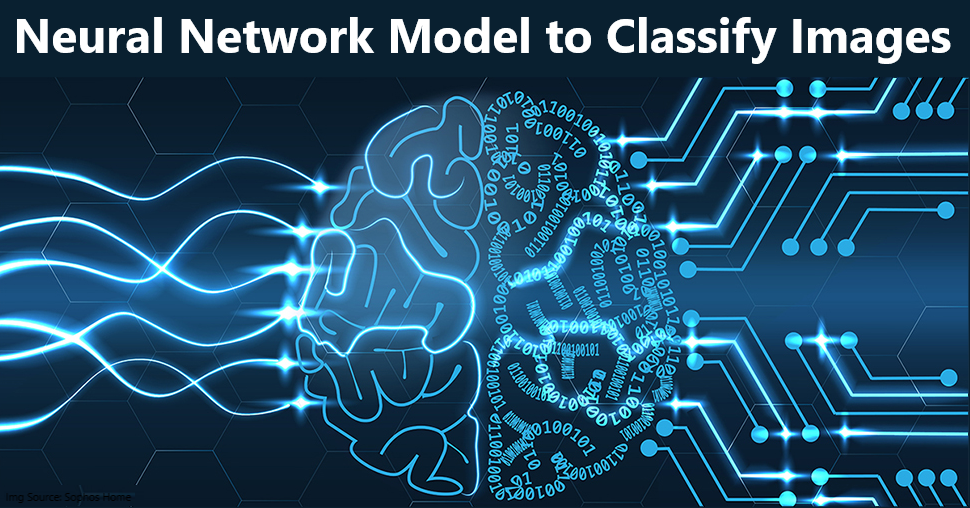# Introduction

In this guide we are going to create and train the neural network model to classify the clothing images. This is based on Basic classification tutorial from TensorFlow. We will use TensorFlow deep learning framework along with Keras high level API to build and train the model.

# Import Libraries

``````import tensorflow as tf
import numpy as np
import matplotlib.pyplot as plt
import os, datetime
``````

## Versions of Imported Libraries

``````import pkg_resources
import types
def get_imports():
for name, val in globals().items():
if isinstance(val, types.ModuleType):
name = val.__name__.split(".")

elif isinstance(val, type):
name = val.__module__.split(".")

if name == "PIL":
name = "Pillow"
elif name == "sklearn":
name = "scikit-learn"

yield name

def get_versions():
imports = list(set(get_imports()))

requirements = []
for m in pkg_resources.working_set:
if m.project_name in imports and m.project_name!="pip":
requirements.append((m.project_name, m.version))

for r in requirements:
print("{}== {}".format(*r))

get_versions()
``````
``````tensorflow== 2.3.0
numpy== 1.18.5
matplotlib== 3.2.1
``````

# List of Files & Devices

``````# List all files under the input directory
for dirname, _, filenames in os.walk('/kaggle/input'):
for filename in filenames:
print(os.path.join(dirname, filename))

# List of physical devices
tf.config.experimental.list_physical_devices()
``````
``````/kaggle/input/fashionmnist/t10k-labels-idx1-ubyte
/kaggle/input/fashionmnist/train-images-idx3-ubyte
/kaggle/input/fashionmnist/fashion-mnist_train.csv
/kaggle/input/fashionmnist/train-labels-idx1-ubyte
/kaggle/input/fashionmnist/t10k-images-idx3-ubyte
/kaggle/input/fashionmnist/fashion-mnist_test.csv
/kaggle/input/fashionmnist-train/fashion-mnist_train.csv

[PhysicalDevice(name='/physical_device:CPU:0', device_type='CPU'),
PhysicalDevice(name='/physical_device:XLA_CPU:0', device_type='XLA_CPU')]
``````

# Load Data

• We are using Fashion MNIST dataset which contains 70,000 grayscale images in 10 categories.
• We will use 60,000 images for training and 10,000 images for testing the model.
• You can load the data directly from TensorFlow using `fashion_mnist.load_data()`
• The images are 28x28 NumPy arrays, with pixel values ranging from 0 to 255. The labels are an array of integers, ranging from 0 to 9. These correspond to the class of clothing the image represents:
Label Class
0 T-shirt/top
1 Trouser
2 Pullover
3 Dress
4 Coat
5 Sandal
6 Shirt
7 Sneaker
8 Bag
9 Ankle boot
• The images show individual articles of clothing at low resolution (28 by 28 pixels), as seen here: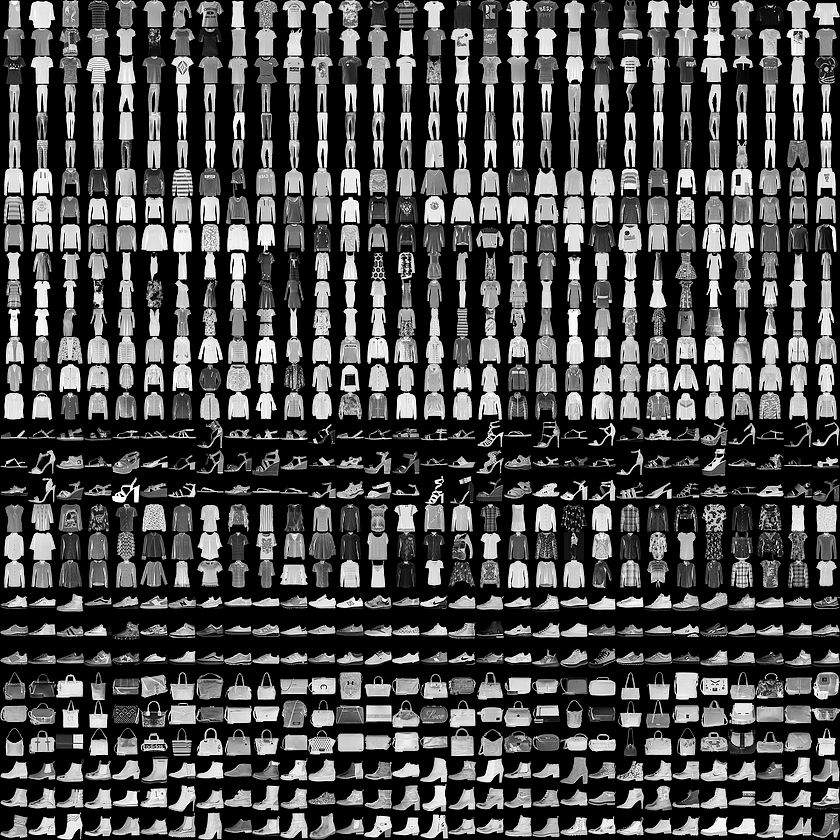``````fashion_mnist = tf.keras.datasets.fashion_mnist
(train_images, train_labels), (test_images, test_labels) = fashion_mnist.load_data()

# Creating class label array
class_names = ['T-shirt/top', 'Trouser', 'Pullover', 'Dress', 'Coat', 'Sandal', 'Shirt', 'Sneaker', 'Bag', 'Ankle boot']
``````
``````Downloading data from https://storage.googleapis.com/tensorflow/tf-keras-datasets/train-labels-idx1-ubyte.gz
32768/29515 [=================================] - 0s 0us/step
Downloading data from https://storage.googleapis.com/tensorflow/tf-keras-datasets/train-images-idx3-ubyte.gz
26427392/26421880 [==============================] - 0s 0us/step
Downloading data from https://storage.googleapis.com/tensorflow/tf-keras-datasets/t10k-labels-idx1-ubyte.gz
8192/5148 [===============================================] - 0s 0us/step
Downloading data from https://storage.googleapis.com/tensorflow/tf-keras-datasets/t10k-images-idx3-ubyte.gz
4423680/4422102 [==============================] - 0s 0us/step
``````

# Exploratory Data Analysis

``````# Shape of training nad test data
print(f'Shape of train_images: {train_images.shape}')
print(f'Shape of train_labels: {train_labels.shape}')
print(f'Shape of test_images: {test_images.shape}')
print(f'Shape of test_labels: {test_labels.shape}')
``````
``````Shape of train_images: (60000, 28, 28)
Shape of train_labels: (60000,)
Shape of test_images: (10000, 28, 28)
Shape of test_labels: (10000,)
``````
``````# There are 10 labels starting from 0 to 9
print(f'Unique train labels: {np.unique(train_labels)}')
print(f'Unique test labels: {np.unique(test_labels)}')
``````
``````Unique train labels: [0 1 2 3 4 5 6 7 8 9]
Unique test labels: [0 1 2 3 4 5 6 7 8 9]
``````

## Data Visualization

``````# The images are 28x28 NumPy arrays, with pixel values ranging from 0 to 255
train_images
``````
``````array([[[0, 0, 0, ..., 0, 0, 0],
[0, 0, 0, ..., 0, 0, 0],
[0, 0, 0, ..., 0, 0, 0],
...,
[0, 0, 0, ..., 0, 0, 0],
[0, 0, 0, ..., 0, 0, 0],
[0, 0, 0, ..., 0, 0, 0]],

[[0, 0, 0, ..., 0, 0, 0],
[0, 0, 0, ..., 0, 0, 0],
[0, 0, 0, ..., 0, 0, 0],
...,
[0, 0, 0, ..., 0, 0, 0],
[0, 0, 0, ..., 0, 0, 0],
[0, 0, 0, ..., 0, 0, 0]],

[[0, 0, 0, ..., 0, 0, 0],
[0, 0, 0, ..., 0, 0, 0],
[0, 0, 0, ..., 0, 0, 0],
...,
[0, 0, 0, ..., 0, 0, 0],
[0, 0, 0, ..., 0, 0, 0],
[0, 0, 0, ..., 0, 0, 0]],

...,

[[0, 0, 0, ..., 0, 0, 0],
[0, 0, 0, ..., 0, 0, 0],
[0, 0, 0, ..., 0, 0, 0],
...,
[0, 0, 0, ..., 0, 0, 0],
[0, 0, 0, ..., 0, 0, 0],
[0, 0, 0, ..., 0, 0, 0]],

[[0, 0, 0, ..., 0, 0, 0],
[0, 0, 0, ..., 0, 0, 0],
[0, 0, 0, ..., 0, 0, 0],
...,
[0, 0, 0, ..., 0, 0, 0],
[0, 0, 0, ..., 0, 0, 0],
[0, 0, 0, ..., 0, 0, 0]],

[[0, 0, 0, ..., 0, 0, 0],
[0, 0, 0, ..., 0, 0, 0],
[0, 0, 0, ..., 0, 0, 0],
...,
[0, 0, 0, ..., 0, 0, 0],
[0, 0, 0, ..., 0, 0, 0],
[0, 0, 0, ..., 0, 0, 0]]], dtype=uint8)
``````
``````
plt.figure()
plt.imshow(train_images)
plt.colorbar()
plt.grid(False)
plt.show()
``````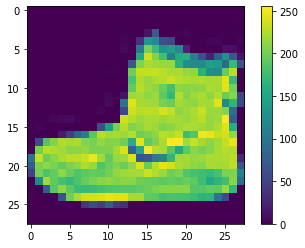``````# Images labels(classes) possible values from 0 to 9
train_labels
``````
``````array([9, 0, 0, ..., 3, 0, 5], dtype=uint8)
``````
``````# Display the first 25 images from the training set and display the class name below each image.
plt.figure(figsize=(10,10))
for i in range(25):
plt.subplot(5,5,i+1)
plt.xticks([])
plt.yticks([])
plt.grid(False)
plt.imshow(train_images[i], cmap=plt.cm.binary)
plt.xlabel(class_names[train_labels[i]])
plt.show()
``````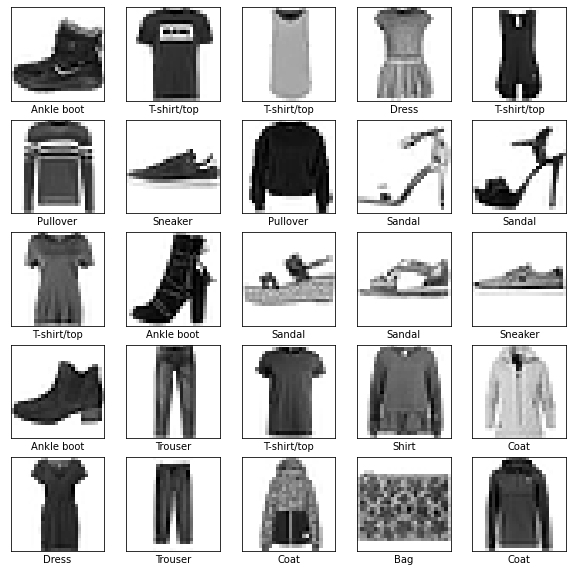# Preprocessing the Data

## Scaling

• Pixel values for each image, fall in the range of 0 to 255.
• Typically zero is taken to be black, and 255 is taken to be white. Values in between make up the different shades of gray.
• In order to scale the input we are going to divide every value by 255 so that final values will be in the range of 0 to 1.
• It’s important that the training set and the testing set be preprocessed in the same way.
``````train_images = train_images / 255.0
test_images = test_images / 255.0
``````

# Model Building

Building the neural network model requires configuring the input, hidden and output layers.

## Set up the Layers

• The basic building block of the neural network is the layer. Layers extract representation from the data fed into them.
• Most times we have to chain multiple layers together to solve the problem.
• The first layer in this network, `tf.keras.layers.Flatten`, transforms the format of the images from a two-dimensional array (of 28 by 28 pixels) to a one-dimensional array (of 28 * 28 = 784 pixels).
• The input layer do not help in any kind of learning, it only reformats the data.
• Once we have flattened input data, we can add dense hidden layers to the network. Here we are using two dense layers.
• The first Dense layer has 128 nodes (or neurons) and using ‘relu’ activation function.
• The second (and last) layer returns a logits array with length of 10. Each node contains a score that indicates the current image belongs to one of the 10 classes. Note that here we are not using any activation function, so by default it will be linear activation function.
``````model = tf.keras.Sequential([
tf.keras.layers.Flatten(input_shape=(28,28)),
tf.keras.layers.Dense(128, activation= 'relu'),
tf.keras.layers.Dense(10) # linear activation function
])
``````

## Compile the Model

• In this step we add all the required settings for the model training.
• Loss Function: To measure models accuracy during training.
• Optimizer: To update the model weights based on the input data and loss function output.
• Metrics: Used to monitor the training the and testing steps
``````# The from_logits=True attribute inform the loss function that the output values generated by the model are not normalized, a.k.a. logits.
# Since softmax layer is not being added at the last layer, hence we need to have the from_logits=True to indicate the probabilities are not normalized.

model.compile(optimizer= 'adam',
loss=tf.keras.losses.SparseCategoricalCrossentropy(from_logits=True),
metrics = ['accuracy'])
``````

## Train the Model

Steps involved in model training are as below

• Feeding the training images and associated labels to the model.
• Model learn the mapping of images and labels.
• Then we ask model to perform predictions using test_images.
• Verify the model predictions using test_labels.

### Feed the Model

• To start training, call the `model.fit` Its called fit because it “fits” the model to the training data.
• As the model trains, the loss and accuracy metrics are displayed. This model reaches an accuracy of about 0.91 (or 91%) on the training data.
``````%%timeit -n1 -r1 # time required toexecute this cell once

# To view in TensorBoard
logdir = os.path.join("logs/adam", datetime.datetime.now().strftime("%Y%m%d-%H%M%S"))
tensorboard_callback = tf.keras.callbacks.TensorBoard(logdir, histogram_freq=1)

model.fit(train_images, train_labels, epochs= 10, callbacks = [tensorboard_callback])
``````
``````Epoch 1/10
1875/1875 [==============================] - 3s 2ms/step - loss: 0.5038 - accuracy: 0.8237
Epoch 2/10
1875/1875 [==============================] - 3s 2ms/step - loss: 0.3757 - accuracy: 0.8644
Epoch 3/10
1875/1875 [==============================] - 3s 1ms/step - loss: 0.3367 - accuracy: 0.8778
Epoch 4/10
1875/1875 [==============================] - 3s 1ms/step - loss: 0.3126 - accuracy: 0.8855
Epoch 5/10
1875/1875 [==============================] - 3s 1ms/step - loss: 0.2963 - accuracy: 0.8912
Epoch 6/10
1875/1875 [==============================] - 3s 1ms/step - loss: 0.2800 - accuracy: 0.8979
Epoch 7/10
1875/1875 [==============================] - 3s 2ms/step - loss: 0.2698 - accuracy: 0.8993
Epoch 8/10
1875/1875 [==============================] - 3s 1ms/step - loss: 0.2593 - accuracy: 0.9041
Epoch 9/10
1875/1875 [==============================] - 3s 2ms/step - loss: 0.2493 - accuracy: 0.9074
Epoch 10/10
1875/1875 [==============================] - 3s 2ms/step - loss: 0.2394 - accuracy: 0.9105
29.8 s ± 0 ns per loop (mean ± std. dev. of 1 run, 1 loop each)
``````

## Model Accuracy

In this step we compare the model’s performance against test data

``````test_loss, test_acc = model.evaluate(test_images, test_labels, verbose= 2)
print(f'\nTest accuracy: {test_acc}')
``````
``````313/313 - 0s - loss: 0.3319 - accuracy: 0.8810

Test accuracy: 0.8809999823570251
``````

As you can notice accuracy on the test dataset is less than the training dataset. This gap between accuracy represent overfitting. For more detail please refer.

## Make Predictions

• We can test the model’s accuracy on few images from test dataset.
• But since our model is using the default ‘linear activation function’ we have to attach a softmax layer to convert the logits to probabilities, which are easier to interpret.
``````probability_model = tf.keras.Sequential([model, tf.keras.layers.Softmax()])
predictions = probability_model.predict(test_images)
``````
``````# 'predictions' will contain the prediction for each image in the training set. Lets check the first prediction
predictions
``````
``````array([1.0144830e-08, 4.8488679e-14, 1.8175688e-11, 5.6300261e-13,
3.1431319e-11, 1.5152204e-03, 1.1492748e-08, 3.7524022e-02,
1.5029757e-07, 9.6096063e-01], dtype=float32)
``````

Since we have 10 nodes in the last layer(one for each lass of image) we get 10 predictions for each image. Each number represents the confidence score for each class of image. We can choose the highest confidence score as final prediction of the model.

``````np.argmax(predictions)
``````
``````9
``````

So the model predict that prediction image represent the 9th index class. `class_names-> ankle boot` Let’s cross-check with true value from test_labels

``````test_labels
``````
``````9
``````

Similarly to verify our predictions for other images, lets write functions that can return prediction, true label along with image.

``````def plot_image(i, predictions_array, true_label, img):
"""
This method will plot the true image and also compare the prediction with true values if matcing write the caption in green color else in red color.
Format is : predicted class %confidence score (true class)

Input:
i: Index of the prediction to test
predictions_array: Every prediction contain array of 10 number
true_label: Correct image labels. In case of test data they are test_labels
img: Test images. In case of test data they are test_images.
"""
true_label, img = true_label[i], img[i]
plt.grid(False)
plt.xticks([])
plt.yticks([])

plt.imshow(img, cmap=plt.cm.binary) # For grayscale colormap

predicted_label = np.argmax(predictions_array)
if predicted_label == true_label:
color = 'green'
else:
color = 'red'

plt.xlabel("{} {:2.0f}% ({})".format(class_names[predicted_label],
100*np.max(predictions_array),
class_names[true_label]),
color=color)

plot_image(0, predictions, test_labels, test_images)
``````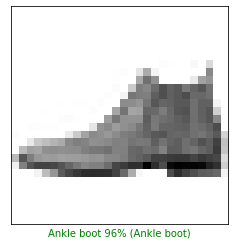Lets write a function that can plot a bar graph for each class prediction.

``````def plot_value_array(i, predictions_array, true_label):
"""
This method will plot the percentage confidence score of each class prediction.

Input:
i: Index of the prediction to test
predictions_array: Every prediction contain array of 10 number
true_label: Correct image labels. In case of test data they are test_labels
"""
true_label = true_label[i]
plt.grid(False)
plt.xticks(range(10))
plt.yticks([])
thisplot = plt.bar(range(10), predictions_array, color="#777777")
plt.ylim([0, 1])
predicted_label = np.argmax(predictions_array)

thisplot[predicted_label].set_color('red')
thisplot[true_label].set_color('green')

i = 0
plot_value_array(i, predictions[i],  test_labels)
``````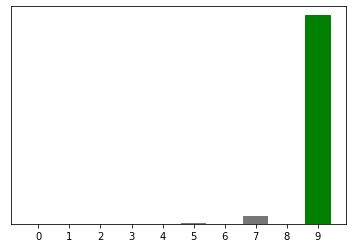Lets try with some random samaple and plot the results for verification.

``````i = 12
plt.figure(figsize=(12,6))
plt.subplot(1,2,1)
plot_image(i, predictions[i], test_labels, test_images)
plt.subplot(1,2,2)
plot_value_array(i, predictions[i],  test_labels)
_ = plt.xticks(range(10), class_names, rotation=45)
plt.show()
``````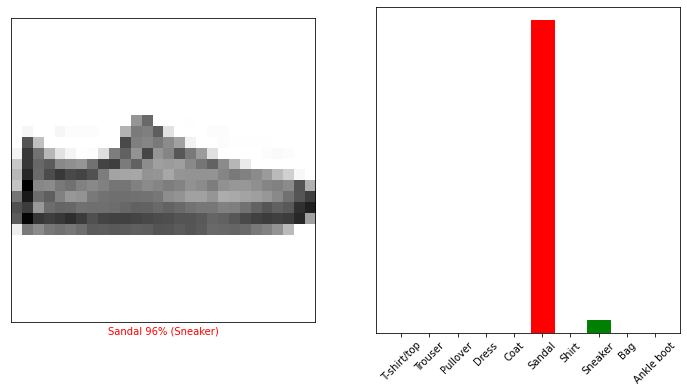As you can see from above result that our prediction for test example 12 is Sandal with confidence score of 83%. But the true label for this prediction is Sneaker. Remember that our models test accuracy is 88% means for 12% predictions will go wrong. In this case since Sandal and Sneaker looks a lot alike, this prediction went wrong. Note that the model can be wrong even when the prediction confidence score is very high!!

Now lets plot few more images and their predictions. We will use the below list for testing. `test_list= [16, 17, 22, 23, 24, 25, 39, 40, 41, 42, 48, 49, 50, 51,66]`

``````# Plot the test images from 'test_list', their predicted labels, and the true labels.
# Color correct predictions in green and incorrect predictions in red.

test_list= [16, 17, 22, 23, 24, 25, 39, 40, 41, 42, 48, 49, 50, 51,66]
num_rows = 5
num_cols = 3
num_images = num_rows * num_cols
plt.figure(figsize=(2*2*num_cols, 2*num_rows))
for i in range(num_images):
plt.subplot(num_rows, 2*num_cols, 2*i+1)
plot_image(test_list[i], predictions[test_list[i]], test_labels, test_images)
plt.subplot(num_rows, 2*num_cols, 2*i+2)
plot_value_array(test_list[i], predictions[test_list[i]], test_labels)
plt.tight_layout()
plt.show()
``````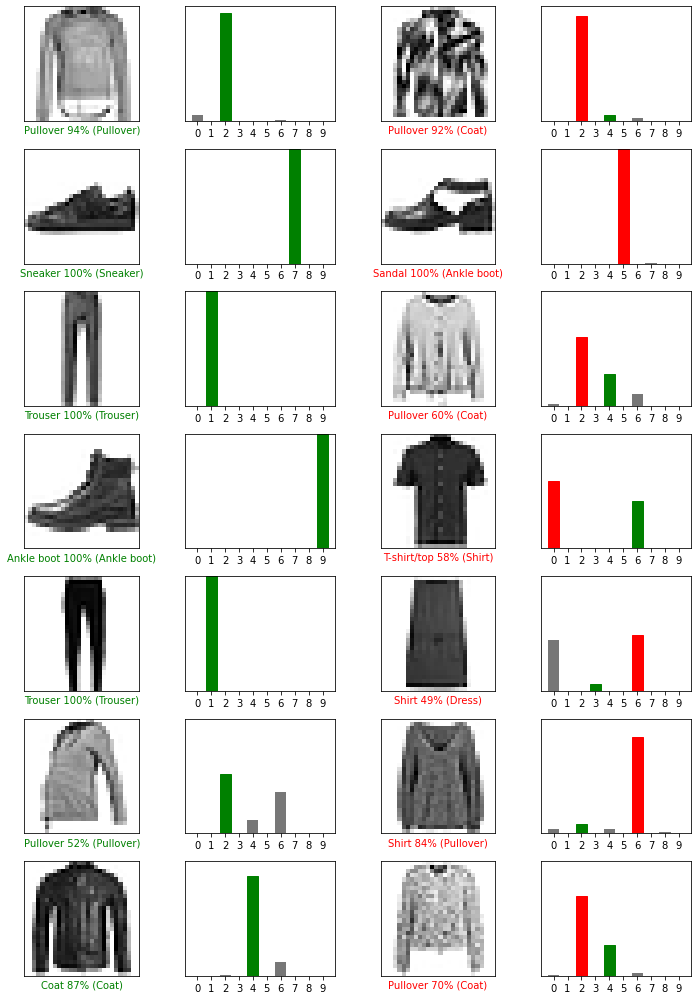# Using the Trained Model

• By default our model is optimized to make predictions on a batch, or collection of example at once.
• We can also use model to make prediction on single image
``````# Grab an image from the test dataset.
img = test_images

print(img.shape)
``````
``````(28, 28)
``````
``````# Add the image to a batch where it's the only member.
img = (np.expand_dims(img, 0))
print(img.shape)
``````
``````(1, 28, 28)
``````

Now predict the correct label for above image (with shape 1, 28, 28)

``````predictions_single = probability_model.predict(img)
# Remember that if we do "predictions = probability_model.predict(test_images)" then we get predictions for all test data"
print(f'Probabilty for all classes: {predictions_single}, \nBest confidence score for class: {np.argmax(predictions_single)}')
``````
``````Probabilty for all classes: [[8.5143931e-03 1.0142570e-05 2.4879885e-01 1.4979002e-03 2.5186172e-02
2.2455691e-09 7.1554321e-01 2.1525864e-11 4.4930150e-04 8.0325089e-09]],
Best confidence score for class: 6
``````

Now lets plot prediction and value array plot for above iamge.

``````i = 49
plt.figure(figsize=(12,6))
plt.subplot(1,2,1)
plot_image(i, predictions_single, test_labels, test_images)
plt.subplot(1,2,2)
plot_value_array(i, predictions_single,  test_labels)
_ = plt.xticks(range(10), class_names, rotation=45)
plt.show()
``````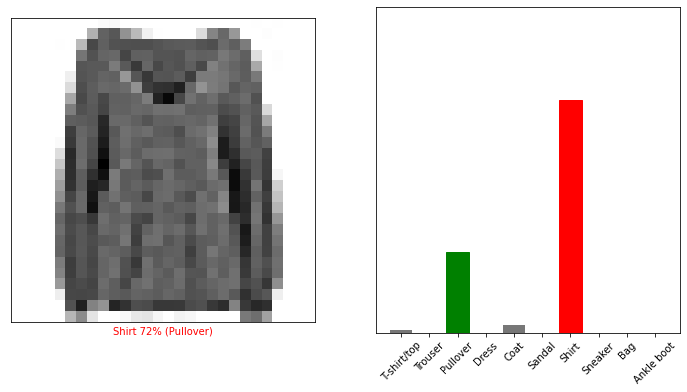## Learning Path for DP-900 Microsoft Azure Data Fundamentals Certification

8 minute read

Learning path to gain necessary skills and to clear the Azure Data Fundamentals Certification. This certification is intended for candidates beginning to wor...

## Learning Path for AI-900 Microsoft Azure AI Fundamentals Certification

7 minute read

Learning path to gain necessary skills and to clear the Azure AI Fundamentals Certification. This certification is intended for candidates with both technica...

## ANN Model to Classify Images

11 minute read

In this guide we are going to create and train the neural network model to classify the clothing images. We will use TensorFlow deep learning framework along...

## Introduction to NLP

8 minute read

In short NLP is an AI technique used to do text analysis. Whenever we have lots of text data to analyze we can use NLP. Apart from text analysis, NLP also us...

## K Fold Cross Validation

13 minute read

There are multiple ways to split the data for model training and testing, in this article we are going to cover K Fold and Stratified K Fold cross validation...

## K-Means Clustering

12 minute read

K-Means clustering is most commonly used unsupervised learning algorithm to find groups in unlabeled data. Here K represents the number of groups or clusters...

## Time Series Analysis and Forecasting

10 minute read

Any data recorded with some fixed interval of time is called as time series data. This fixed interval can be hourly, daily, monthly or yearly. Objective of t...

## Support Vector Machines

9 minute read

Support vector machines is one of the most powerful ‘Black Box’ machine learning algorithm. It belongs to the family of supervised learning algorithm. Used t...

## Random Forest

11 minute read

Random forest is supervised learning algorithm and can be used to solve classification and regression problems. Unlike decision tree random forest fits multi...

## Decision Tree

14 minute read

Decision tree explained using classification and regression example. The objective of decision tree is to split the data in such a way that at the end we hav...

## Agile Scrum Framework

7 minute read

This tutorial covers basic Agile principles and use of Scrum framework in software development projects.

## Underfitting & Overfitting

2 minute read

Main objective of any machine learning model is to generalize the learning based on training data, so that it will be able to do predictions accurately on un...

## Multiclass Logistic Regression Using Sklearn

6 minute read

In this study we are going to use the Linear Model from Sklearn library to perform Multi class Logistic Regression. We are going to use handwritten digit’s d...

## Binary Logistic Regression Using Sklearn

5 minute read

In this tutorial we are going to use the Logistic Model from Sklearn library. We are also going to use the same test data used in Logistic Regression From Sc...

## Logistic Regression From Scratch With Python

13 minute read

This tutorial covers basic concepts of logistic regression. I will explain the process of creating a model right from hypothesis function to algorithm. We wi...

## Train Test Split

3 minute read

In this tutorial we are going to study about train, test data split. We will use sklearn library to do the data split.

## One Hot Encoding

11 minute read

In this tutorial we are going to study about One Hot Encoding. We will also use pandas and sklearn libraries to convert categorical data into numeric data.

## Multivariate Linear Regression Using Scikit Learn

8 minute read

In this tutorial we are going to use the Linear Models from Sklearn library. Scikit-learn is one of the most popular open source machine learning library for...

## Univariate Linear Regression Using Scikit Learn

7 minute read

In this tutorial we are going to use the Linear Models from Sklearn library. Scikit-learn is one of the most popular open source machine learning library for...

## Multivariate Linear Regression From Scratch With Python

10 minute read

In this tutorial we are going to cover linear regression with multiple input variables. We are going to use same model that we have created in Univariate Lin...

## Univariate Linear Regression From Scratch With Python

13 minute read

This tutorial covers basic concepts of linear regression. I will explain the process of creating a model right from hypothesis function to gradient descent a...

## Machine Learning Introduction And Learning Plan

4 minute read

In this tutorial we will see the brief introduction of Machine Learning and preferred learning plan for beginners

Back to top ↑